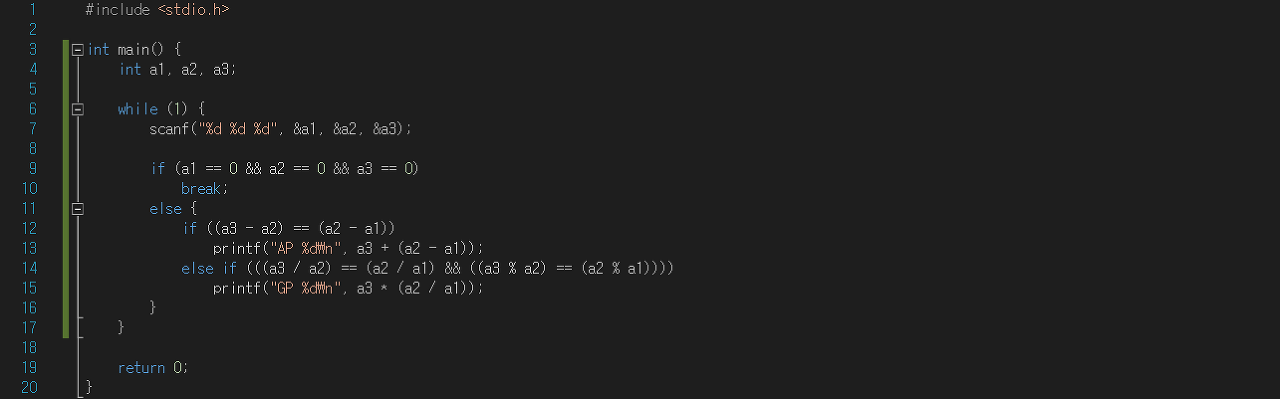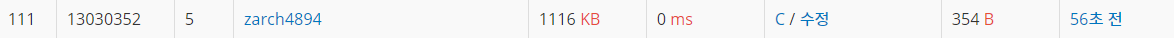[백준] 4880 - What's Next?문제 정보

• 문제 이름: What’s Next? (원문)
• 문제 번호: 4880
• 문제 유형: -
• 풀이 언어: C
• 제출 시간: 2019년 5월 4일 17시 25분 44초
• 원본 링크: https://www.acmicpc.net/problem/4880

문제

According to Wikipedia, an arithmetic progression (AP) is a sequence of numbers such that the diﬀerence of any two successive members of the sequence is a constant. For instance, the sequence 3, 5, 7, 9, 11, 13, . . . is an arithmetic progression with common diﬀerence 2. For this problem, we will limit ourselves to arithmetic progression whose common diﬀerence is a non-zero integer.

On the other hand, a geometric progression (GP) is a sequence of numbers where each term after the ﬁrst is found by multiplying the previous one by a ﬁxed non-zero number called the common ratio. For example, the sequence 2, 6, 18, 54, . . . is a geometric progression with common ratio 3. For this problem, we will limit ourselves to geometric progression whose common ratio is a non-zero integer.

Given three successive members of a sequence, you need to determine the type of the progression and the next successive member.

입력

Your program will be tested on one or more test cases. Each case is speciﬁed on a single line with three integers (−10,000 < a1, a2, a3 < 10,000) where a1, a2, and a3 are distinct. The last case is followed by a line with three zeros.

출력

For each test case, you program must print a single line of the form: XX┐ v

where XX is either AP or GP depending if the given progression is an Arithmetic or Geometric Progression. v is the next member of the given sequence. All input cases are guaranteed to be either an arithmetic or geometric progressions.

결과 - 소스코드 및 랭크메모

1년 전에 틀렸던 문제였는데 오늘 다시 시도해보았다.

전에 이 문제를 풀 때 분명 쉬운 문제인데 왜 안 풀리지 고민을 하면서 여러 번 제출을 했었는데 이제와서 다시 보니 등비수열을 이루는 부분에서 몫만 신경쓰고 나머지 값은 생각하지 않고 있었던 것이었다.

그래서 그 경우를 추가하여 문제를 해결할 수 있었다.

Alec J

2019-05-04

2021-02-09